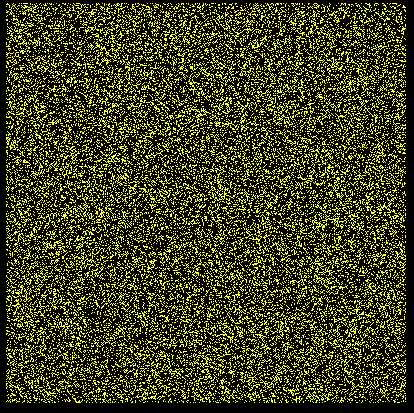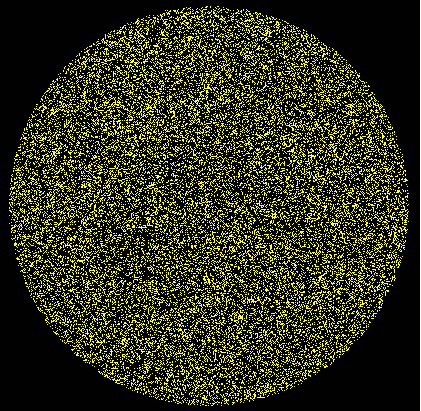# π, and finding it at random!

## ( via circles, cubes, spheres and hypervolumes)- -Like most school kids I met π in maths classes about circles- and then spheres. Any teenager should know 'two π r ' ( circumference), and most will know 'π r squared' ( area), and probably 'four thirds π r cubed' ( volume). And they'll probably have seen approximations to circle areas via radii and polygons. Then they may meet various approximations, from numerological conveniences like 22/7 to a succession of power series. I've been able to quote the first ten digits of π ever since- although when first learned we used four-figure logs ( or slide rules), and few measurements justified high accuracy.

Most of the simpler power series converge too slowly to be of much use- and our maths teachers regaled us with stories of early mathematicians doggedly calculating by hand to increasing number of significant figures- and often later being found to have made an error somewhere. A bit like the medieval counting of how many angels can stand on the head of a needle...

It was after that stage that I came across the idea that you can approximate π via 'stochastic' ( ie random) methods. The two usual ones mentioned are the 'dartboard' method ( where you throw lots of darts at random at a circular target), and Bouffon's 'needle' ( where you drop huge numbers of identical rods on a space ruled with parallel lines and count the fraction that cross a line out of the total thrown number.)

The maths of the 'dartboard' method are trivial. Inscribe a circle of radius one in a square of side two. Throw ( lots!) of random darts at ithe general area. Count what number fell inside the square, and also those which lay inside only the circle. Ignore any which totally missed. Since the circle area /square area = π/ 4, multiply the result by four and you have an approximation to π. Not especially accurate, and even at computer machine code speeds not a good way to get more than a few tens of digits!- -If we move up to 3D, we are throwing random darts into a 3D cube, and finding how many lie in a spherical volume. The ( 2D) projection of the spherical 'inside' volume no longer has a sharp edge, since it can be thought of as the sum of many layers, and only the one going through the origin has the full unit radius.- -This becomes increasingly obvious if we move on up to more dimensions- but can still be used to approximate pi.

If you really want more accurate digits for pi, use one of yhe power series.

## LB code for circle, sphere and hyperspherical volumes

```
'   *************************************************************************************
'   **
'   **   Pi from crcles, spheres  and hyperspheres.    tenochtitlanuk    March 2018    **
'   **
'   *************************************************************************************

'   Throw random coordinates in a figure of side 2 ( +1 >x >-1) ( think of darts thrown at a dartboard)

'   The positions thrown into occupy (hyper)volume of 2^number of dimensions.
'       This specifies a volume of 2^numberOfDimensions ( eg in 3D cube 2x2x2 =8 m^3.

'   If each one is within ( x^2 +y^2 +...)^0.5 <1 you are within a circle/ sphere/ hypersphere....
'       with volume pi r^2,  4/3 pi r^3. 1/2 pi^2 r^4, etc. ( and r =1 by choice.)

'   Ratio of ( inside /total) is pi /4, 2 *pi/3, ( pi^2 /32)^0.5,  etc

'   Easy to display in 2D. Harder in TRUE ( projected) 3D. Never did succeed in 4D!
'   Did see 2D screen display of 4D hypercube rotating on any axis- on an analogue computer..
'       ( But of course easy to show 2D projection for any number of dimensions over 2...)

'   _____________________________________________________________________________________________

nomainwin

WindowWidth  =430
WindowHeight =500

open "Pi finder" for graphics_nsb as #wg

#wg "trapclose [quit]; down"

N               =100000         '   number of throws

'spaceDimensions = number of space dimensions to consider ( positive, integer)
'       physicist?      -choose 2 <= space_Dims =<11
'       mathematician?  -anything you like!

'dim Space( spaceDimensions)     '   Space( 1) represents x; space( 2) is y; etc....
'    only needed for runs with dimensions > ( default) 10.

for spaceDimensions =2 to 10
inside =0
#wg "fill black ; flush"
#wg "up ; goto 40 450 ; down ; backcolor black ; color white ; font 16"
#wg "\"; str\$( spaceDimensions) +"-space"

for i =1 to N
Dsquared =0

for j =1 to spaceDimensions
Space( j) =2 *rnd( 1) -1
Dsquared =Dsquared +Space( j)^2
next j

if Dsquared >1 then
#wg "color 0 0 0"
else
#wg "color 255 255 60"
inside =inside +1
end if

#wg "set "; 210 +200 *Space( 1); " "; 210 +200 *Space( 2)

scan
next i

#wg "up ; goto 40 450 ; down ; backcolor black ; color white"
#wg "font 16"

#wg "up ; goto 200 450 ; down"

if spaceDimensions =2 then #wg "\ "; str\$( inside /N *4)                   '   for 2D
if spaceDimensions =3 then #wg "\ "; str\$( inside /N *6)                   '   for 3D
if spaceDimensions =4 then #wg "\ "; str\$( ( 32 *inside /N)^0.5)           '   for 4D
if spaceDimensions =5 then #wg "\ "; str\$( ( 15 *32 /8 *inside /N)^0.5)    '   for 5D
if spaceDimensions =6 then #wg "\ "; str\$( ( 6 *64 *inside /N)^0.33333333)           '   for  6D
if spaceDimensions =7 then #wg "\ "; str\$( ( 105 *128 /16 *inside /N)^0.33333333)    '   for  7D
if spaceDimensions =8 then #wg "\ "; str\$( ( 24 /32 *inside /N)^0.25)                '   for  8D
if spaceDimensions =9 then #wg "\ "; str\$( ( 945 /32 /64 *inside /N)^0.25)           '   for  9D
if spaceDimensions =10 then #wg "\ "; str\$( ( 120 /128 *inside /N)^0.2)               '   for 10D

#wg "flush"
#wg "getbmp scr 0 0 430 500"
bmpsave "scr", str\$( spaceDimensions) +"_D.bmp"

timer 1000, [g]
wait
[g]
timer 0

next spaceDimensions

wait

function coefficient( s)
select case s
case 1
c =pi
case 2
c =4 /3 *pi
case else
c =2 *pi /n *coefficient( s -2)
end select

coefficient =c
end function

[quit]
close #wg
end
```